# Variation of a Function

## Variation of a Function

one of the most important characteristics of a function of a real variable. Let f (x) be a function defined on some interval [a, b], the least upper bound of the sums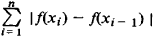over all subdivisions

a =x0 < x1 < . . . < x n-1 < xn = b

of the interval [a, b] is called the total variation of fix) on [a, b]. The geometric sense of the total variation of a continuous function f (x) is the length of the projection of the curve y = f(x) to the.y-axis, with multiple coverings taken into consideration (Banach’s theorem). The total variation of a function f (x) on a segment [a, b] is usually denoted by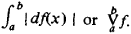If the function f(x) has a continuous derivative, then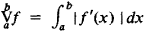Other properties of the variation of a function are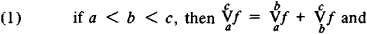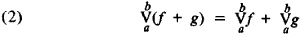There exist continuous functions whose total variation is infinite. One such function is y = x sin (IA) on the interval [—1, 4–1]. If the total variation of a function is finite, then such a function is called a function of bounded variation. Functions of bounded variation were first defined and studied by C. Jordan (1881). Many important functions are of bounded variation—for example, monotonic functions given on an interval, functions with a finite number of maxima and minima, and functions that satisfy a Lipschitz condition. A function of bounded variation on an interval [a, b] has at most countably many discontinuities, the discontinuities are necessarily jump discontinuities, and the function is Riemann integrable and can be represented as the difference of two nondecreasing functions (Jordan). The limit of a converging sequence of functions of bounded variation whose total variations have a common bound is a function of bounded variation. Functions of bounded variation have almost everywhere a finite derivative that is Lebesgue integrable (H. Lebesgue’s theorem).

Functions of bounded variation have applications in the theory of Stieltjes integrals, in the theory of trigonometric series, and in geometry.

### REFERENCES

Aleksandrov, P. S., and A. N. Kolmogorov. Vvedenie v teoriiu funktsii deistvitel’nogo peremennogo, 3rd ed. Moscow-Leningrad, 1938.
Kamke, E. Integral Lebega-Stilt’esa. Moscow, 1959. (Translated from German.)